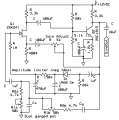# How to control the amplitude of output voltage in wien bridge oscillator?

#### wldb737

Joined May 6, 2018
3
I want to change the amplitude of output voltage in wien bridge oscillator depending on the value of sensor. (maybe pressure sensor)
What element decide the amplitude of output????

#### DickCappels

Joined Aug 21, 2008
6,300
It depends on how the amplitude is stabilized. There is more than one method.

#### wldb737

Joined May 6, 2018
3
It depends on how the amplitude is stabilized. There is more than one method.

This is my circuit.
Depending on what switch is closed, ouput voltage's frequency is changed.
Then, how can I change the amplitude??
Is there any way to change amplitude using variable voltage? (pressure sensor's output is voltage)

#### DickCappels

Joined Aug 21, 2008
6,300
The output amplitude is function of power supply voltage and opamp characteristics.

You could try controlling the amplitude by varying the power supply voltage, or you could try a circuit like the one below,, in which case you would put a resistor in series with the anode of D1 and sum current into the anode of D1 through another resistor to modify the ampltude.The cleanest solution is probably to pass the output of the unmodified oscillator through a gain control circuit such as a transconductance multiplier or multiplying DAC.
(pdf) MT-079: Analog Multipliers

Joined Mar 10, 2018
4,057

#### wldb737

Joined May 6, 2018
3
The output amplitude is function of power supply voltage and opamp characteristics.

You could try controlling the amplitude by varying the power supply voltage, or you could try a circuit like the one below,, in which case you would put a resistor in series with the anode of D1 and sum current into the anode of D1 through another resistor to modify the ampltude.

View attachment 151985